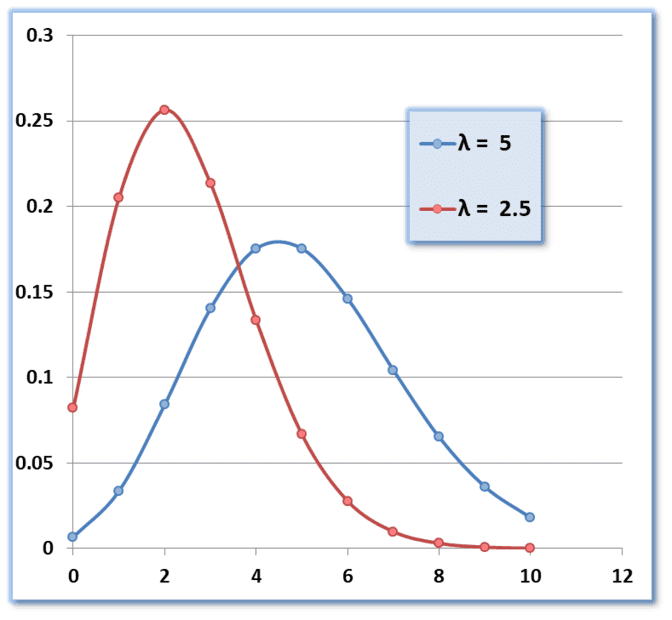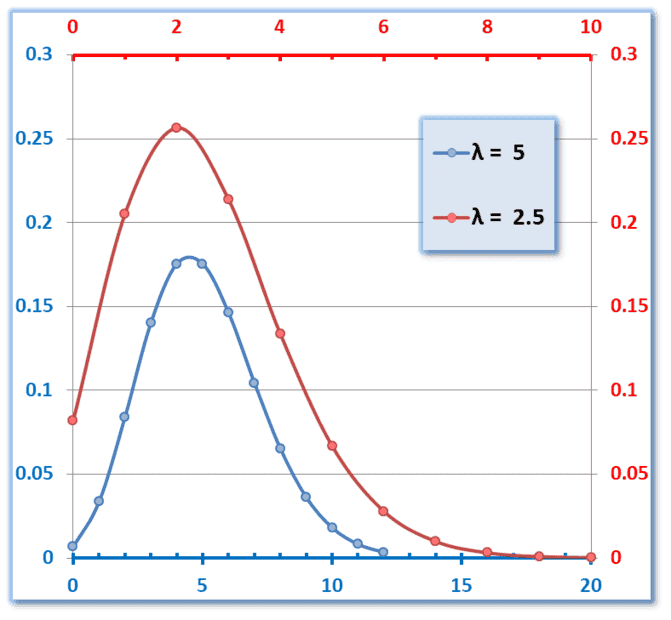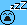# Need help with a Poisson distribution

• barryj

#### barryj

Homework Statement
On average, there are 5 dogs in 100 square miles . What is the probability of 2 dogs in 50 square miles.
Relevant Equations
P(X =x) = e^(-lambda) * ( lambda^x/x!) This is the poisson probability eq.
Please excuse me for posting in this group.l There seems to be little activity in the statistics group and i might get no response.

For this example, lambda, is the average of dogs per 100 square miles = 0.05.
if I wanted the probability of 2 dogs in 100 square miles I would calculate..

P(x=2) = e^(-.05) * (0.05^2/2!) = 0.00119

I assume that for the probability of 2 dogs in a 50 square mile area the lambda would be the same, i.e. 5 in 100 square miles would be the same as 2.5 in 50 square miles = 0.05 and the probability would be the same as above.

Where is my thinking wrong?

For this example, lambda, is the average of dogs per 100 square miles = 0.05.
this contradicts the problem statement, where it clearly says 5, not 0.05So for a 100 square mile area lambda is 5.
For an area half that size, 2.5 might be a good lambda to insert in your formula, don't you think ?

##\ ##

I see your point so the probability of 2 dogs in 100 sq miles would be..

e^(-5) * ( 5^2/2! )= 0.08422

and the probability of 2 dogs in a 50 sq mile areas would be e^(-2.5) *( 2.5^2/2!) = 0.256

Doesn't seem to make sense to me ? The probability of a dog is larger in the smaller area?
What am I missing here

No, the probability of exactly two dogs is larger in the smaller area. Try plotting P(n) vs. n for, say, n = 0 to 10, for both 100 and 50 square miles. Does the difference in the probability distributions make sense?

No, the probability of exactly two dogs is larger in the smaller area.

If this is true, then if I had a tiny area I would have a great probability of 2 dogs. Does not make sense to me. I would think that the bigger area would have a greater probability of dogs.

I will graph the distribution and see how it looks.
I am confused.

Last edited:
No, the probability of exactly two dogs is larger in the smaller area.

If this is true, then if I had a tiny area I would have a great probability of 2 dogs. Does not make sense to me. I would think that the bigger area would have a greater probability of dogs.
No. To maximize the probability of getting exactly two dogs, the area needs to be large enough for an increased probability of two dogs but small enough for a decreased probability of getting more than two dogs

I think I am getting it but to be clear. For the case of Prob of 2 dogs in 50 square miles, the lambda should be 2.5. Is this correct.

I plotted the distribution of both cases and the 50 square mile probability peaked at 2 while the probability of the 100 square mile peaked at about 4.5. This shows that the probability of two dogs in a smaller area is greater.

I guess math does not lie.

I think I am getting it but to be clear. For the case of Prob of 2 dogs in 50 square miles, the lambda should be 2.5. Is this correct.
Yes. ##\lambda## is the expected number of occurrences in a certain time (or area). You are given the expected number of dogs in 100 miles (5 dogs) (##\lambda_{100}=5##), but you are interested 50 miles (##\lambda_{50}=?##). The expected number of dogs in 50 miles is 50/100 * 5 = 2.5. So 2.5 is that value of ##\lambda_{50}## that you should use.
I plotted the distribution of both cases and the 50 square mile probability peaked at 2 while the probability of the 100 square mile peaked at about 4.5. This shows that the probability of two dogs in a smaller area is greater.
Also, notice that the ##\lambda## for 50 miles (##\lambda_{50}=2.5##) is much closer to 2 than the ##\lambda## value for 100 miles (##\lambda_{100}=5##). So it is intuitive that the probability for 2 dogs would be greater for 50 miles.

Last edited:
I think this topic will keep me awake tonight :-)

•BvU
I think I am getting it but to be clear. For the case of Prob of 2 dogs in 50 square miles, the lambda should be 2.5. Is this correct.

I plotted the distribution of both cases and the 50 square mile probability peaked at 2 while the probability of the 100 square mile peaked at about 4.5. This shows that the probability of two dogs in a smaller area is greater.

I guess math does not lie.
Intriguing, isn't it ?
I also followed @mjc123 's advice -- and also manipulated the x-axes a little bit:On the left: one horizontal axis. Both plots have area 1 .
On the right, the plot for ##\ \lambda=2.5\ ## has the red x-axis stretched by a factor 2.

So yes, the probability to find exactly 2 dogs in 50 square miles is higher than the probability to find exactly 5 dogs in 100 square miles.

And outside the 'peak' the factors are even higher:
1 dog in 50 sq mi has 20.5 %, but two in 100 has only 8.4 % and
5 dogs in 50: 6.7% but 10 dogs in 100 only 1.8%

As you state (not: guess!) : the math does not lie.
And it makes sense. In your exercise the area is halved. Would be nice to repeat, but now with 100 sq mi and e.g. 1000##\ ##

This looks a bit fishy, at a P = 10, the 2.5 case is higher on one graph but not on the other?
In fact the 2.5 case always seems to be higher for some reason. The graphs are not the same , are they?

 λ = λ = 5​ 2.5​ 0​ 0.006738​ 0.082085​ 1​ 0.03369​ 0.205212​ 2​ 0.084224​ 0.256516​ 3​ 0.140374​ 0.213763​ 4​ 0.175467​ 0.133602​ 5​ 0.175467​ 0.066801​ 6​ 0.146223​ 0.027834​ 7​ 0.104445​ 0.009941​ 8​ 0.065278​ 0.003106​ 9​ 0.036266​ 0.000863​ 10​ 0.018133​ 0.000216​ 11​ 0.008242​ 4.9E-05​ 12​ 0.003434​ 1.02E-05​ summed 0.968172​ 0.999723​

I get it. I was mis reading the axis.

•BvU
This problem is hard to visualize. Let me explain my issue.

take the case of 5 dogs in100 square feet.

If I walk around the property there is some probability that I would encounter 2 dogs.
But if I roped off half of the property and walked around the roped off section, it doesn't seem likely that the probability of encountering 2 dogs would be greater in the roped off area..

That is indeed a huge change of scale! I don't even know how many square feet fit in a square furlong mileWith on average 5 dogs per 100 sq mi in e.g. a state like Ohio, the probability of having 2 dogs in 100 sq miles is 8.4 %, in 50 sq miles it is 25.6 %, so three times higher (third line in the table).
Get some sleep (I will) and try again tomorrow !

##\ ##

I also calculated the probabilities and got the same answers as you did in your table.

This problem is hard to visualize. Let me explain my issue.

take the case of 5 dogs in100 square feet.

If I walk around the property there is some probability that I would encounter 2 dogs.
But if I roped off half of the property and walked around the roped off section, it doesn't seem likely that the probability of encountering 2 dogs would be greater in the roped off area..
In 100 ft, you expect 5 dogs, not 2. Are you sure that the possibility of encountering 3 dogs or more is not enough to lower the 100 square foot probability of encountering EXACTLY two dogs?
Remember that the expected average in 50 ft is 2.5, so why wouldn't EXACTLY 2 dogs be more likely there than if you had 100 ft, where the expected number is 5 dogs?
My first reaction when reading your original post was that the problem statement was not clear. Is it talking about finding EXACTLY 2 dogs, or about finding 2 OR MORE dogs. The wording of the problem does not make it clear. You seem to be thinking that it is asking about 2 OR MORE dogs.

Last edited: